1. Introduction

It is often necessary to create graphs to effectively communicate key patterns within a dataset. While many software packages allow the user to make basic plots, it can be challenging to create plots that are customized to address a specific idea. While there are numerous ways to create graphs, this tutorial will focus on the R package ggplot2, created by Hadley Wickham.

There are two key functions that are used in ggplot2:

• qplot()or quick plot is similar to base plotting functions in R and is primarily used to produce quick and easy graphics.
• ggplot()the grammar of graphics plot is different from other graphics funcitons because it uses a particular grammar inspired by Leland Wilkinson’s landmark book, The Grammar of Graphics, that focused on thinking about, reasoning with and communicating with graphics. It enables layering of independent components to create custom graphics.
# This tutorial will use the following two packages
library(ggplot2)
library(mosaic)
library(manipulate)

Data: In this tutorial, we will use the AmesHousing data, which provides information on the sales of individual residential properties in Ames, Iowa from 2006 to 2010. The data set contains 2930 observations, and a large number of explanatory variables involved in assessing home values. A full description of this dataset can be found here.

# The csv file should be imported into rstudio:
# str(AmesHousing)

2. The qplot function

In this section, we will briefly provide examples of how the qplot function can be used to create basic graphs. Run the code below and answer Questions 1)-5).

# Create a histogram of housing prices
qplot(data=AmesHousing, x=SalePrice, main ="Histogram of Housing Prices in Ames, Iowa")
# Create a scatterplot of above ground living area by sales price
qplot(data=AmesHousing,x=Gr.Liv.Area, y=SalePrice)
# Create a scatterplot with log transformed variables, coloring by a third variable
qplot(data=AmesHousing,x=log(Gr.Liv.Area),y=log(SalePrice),color=Kitchen.Qual)
# Create distinct scatterplots for each type of kitchen quality and number of fireplaces
qplot(data=AmesHousing,x=Gr.Liv.Area,y=SalePrice,facets=Kitchen.Qual~Fireplaces)
# Create a dotplot of sale prices by kitchen quality
qplot(data=AmesHousing,x=Kitchen.Qual,y=SalePrice)
# Create a boxplot of sale prices by kitchen quality
qplot(data=AmesHousing,x=Kitchen.Qual,y=log(SalePrice),geom="boxplot")

Questions:

1. In this dataset, how many houses were sold with four fireplaces?
2. What is the facet argument used for?
3. Based upon the data documentation, what are the five different levels for kitchen quality?
4. Do these graphs indicate that the quality of a kitchen could be related to the sale price?
5. In the RStudio console, type ?qplot. Modify the above code to create a barchart (geom=bar) of sales by kitchen quality.

3. The basic structure of the ggplot function

All ggplot functions must have at least three components:

• data frame: In this activity we will be using the AmesHousing data.
• geom: to determine the type of geometric shape used to display the data, such as line, bar, point, or area.
• aes: to determine how variables in the data are mapped to visual properties (aesthetics) of geoms. This can include x position, y position, color, shape, fill, and size.

Thus the simplest code for a graphic made with ggplot() would have one of the the following forms:

• ggplot(data, aes(x, y)) + geom_line() or
• ggplot(data) + geom_line(aes(x, y)).

Note the two lines of code above would provide identical results. In the first case, the aes is set as the default for all geoms. In essense, the same x and y variables are used throughout the entire graphic. However, as graphics get more complex, it is often best to creating local aes mappings for each geom as shown in the second line of code.

# Create a histogram of housing prices
ggplot(data=AmesHousing) + geom_histogram(mapping = aes(SalePrice))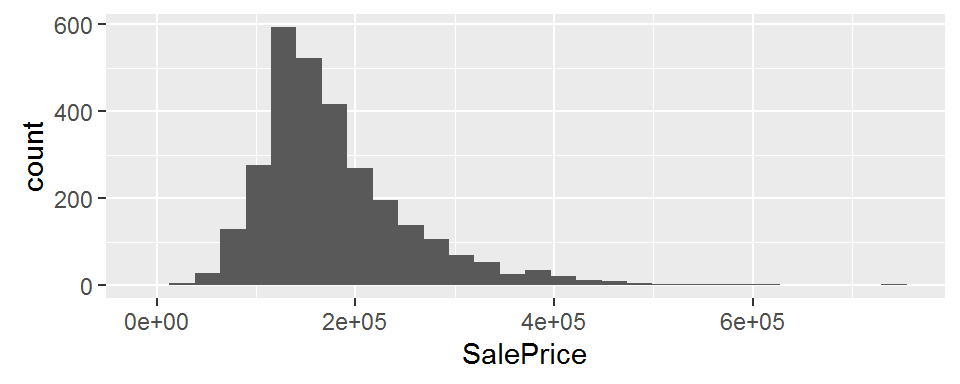In the above code, the terms data= and mapping= are not required, but are used for clarification. For example, the following code will produce identical results:
ggplot(AmesHousing) + geom_histogram(aes(SalePrice)).

# Create a scatterplot of above ground living area by sales price
ggplot(data=AmesHousing) + geom_point(mapping= aes(x=Gr.Liv.Area, y=SalePrice))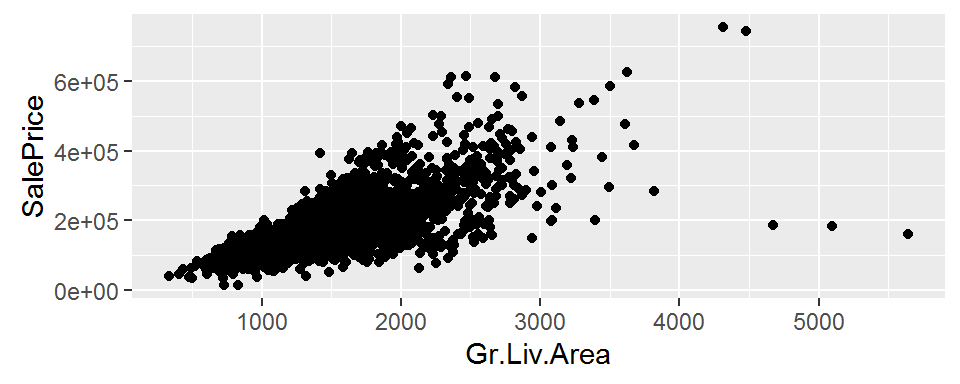Questions:

1. Modify the code for histogram above so that the aes is not within the geom. However the resulting graph should look identical to the one above.
2. Create a scatterplot using ggplot with Fireplaces as the x-axis and SalePrice as the y-axis.

4. Customizing graphics using the ggplot function

In the following code, we layer additional components onto the two graphs shown above.

ggplot(data=AmesHousing) +
geom_histogram(mapping = aes(SalePrice/100000),
breaks=seq(0, 7, by = 1), col="red", fill="lightblue") +
geom_density(mapping = aes(x=SalePrice/100000, y = (..count..)))  +
labs(title="Figure 9: Housing Prices in Ames, Iowa (in \$100,000)",
x="Sale Price of Individual Homes")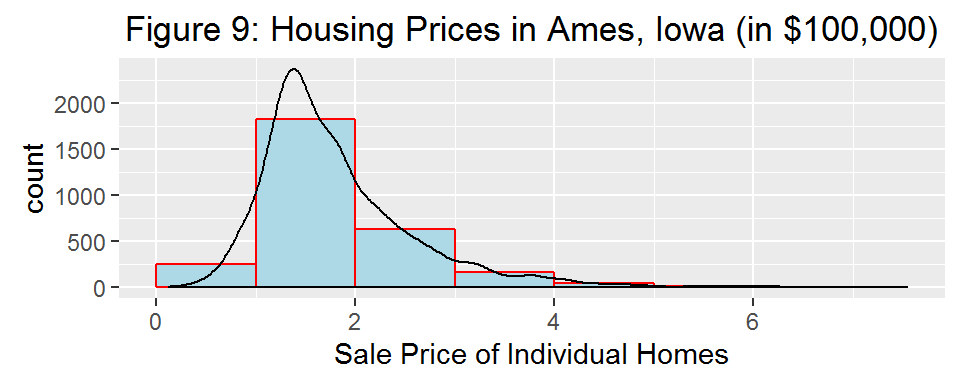Remarks:

• The histogram geom transforms the SalePrice, modifies the bin size and changes the color.
• geom_density overlays a density curve on top of the histogram.
• Typically density curves and histrograms have very different scales, here we use y = (..count..) to modify the density. Alternatively, we could specify aes(x = SalePrice/100000, y = (..density..)) in the histogram geom.
• The labs() command adds a title and an x-axis label. A y-axis label can also be added by using y = " “.

In the code below we create three scatterplots of the log of the above ground living area by the log of sales price

ggplot(data=AmesHousing, aes(x=log(Gr.Liv.Area), y=log(SalePrice)) ) +
geom_point(shape = 3, color = "darkgreen") +
geom_smooth(method=lm,  color="green") +
labs(title="Figure 10: Housing Prices in Ames, Iowa")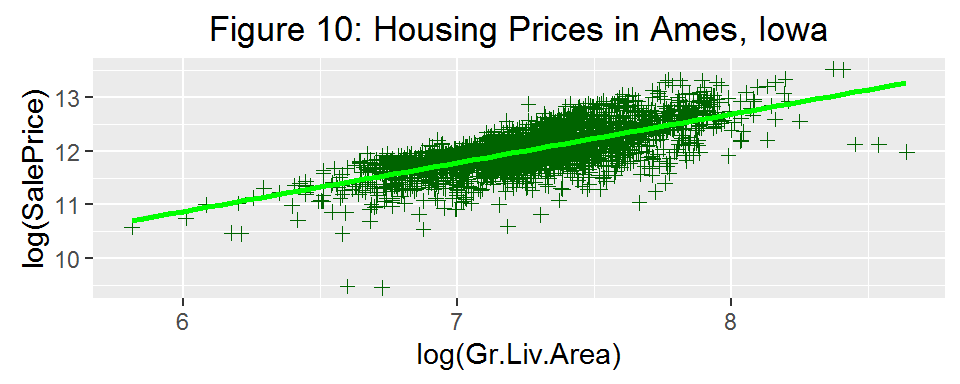ggplot(data=AmesHousing) +
geom_point(aes(x=log(Gr.Liv.Area), y=log(SalePrice), color=Kitchen.Qual),shape=2, size=2) +
geom_smooth(aes(x=log(Gr.Liv.Area), y=log(SalePrice), color=Kitchen.Qual),
method=loess, size=1) +
labs(title="Figure 11: Housing Prices in Ames, Iowa")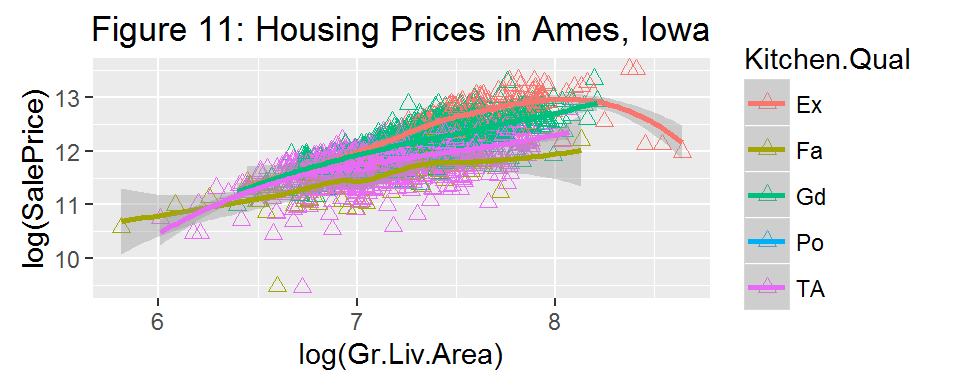ggplot(data=AmesHousing) +
geom_point(mapping = aes(x=log(Gr.Liv.Area), y=log(SalePrice), color=Kitchen.Qual)) +
geom_smooth(mapping = aes(x=log(Gr.Liv.Area), y=log(SalePrice), color=Kitchen.Qual),
method=lm, se=FALSE, fullrange=TRUE) +
facet_grid(. ~ Fireplaces) +
labs(title="Figure 12: Housing Prices in Ames, Iowa")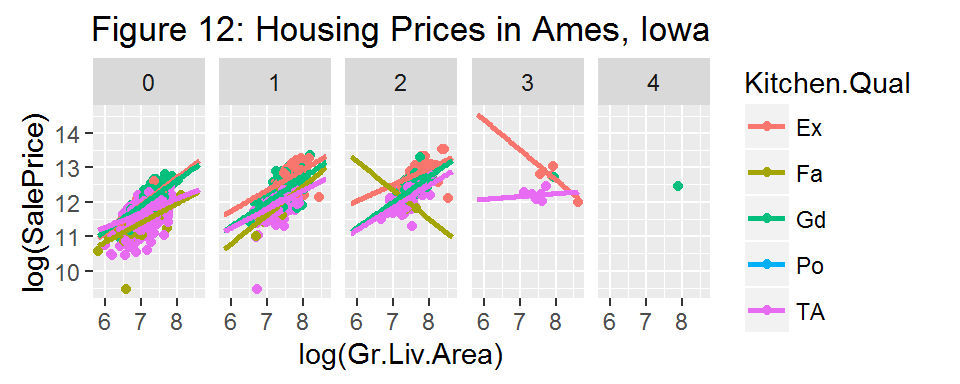Remarks:

• geom_point is used to create a scatterplot. As shown in Figure 10, multiple shapes can be used as points. The Data Visualization Cheat Sheet lists several shape options
• geom_smooth adds a fitted line through the data.
• method=lm specifies a linear regression line. method=loess in the creates a smooth fit curve.
• se=FALSE removes the shaded confidence regions around each line.
• fullrange=TRUE extends all regression lines to the same length
• facet_grid and facet_wrap commands are used to create multiple plots. In Figure 12, we have created separate scatterplots based upon the number of fireplaces.
• When assigning fixed characteristics, (such as color, shape or size), the commands occur outside the aes, as in Figure 10, color="green". When characteristics are dependent on the data, the command should occur within the aes, such as in Figure 11 color=Kitchen.Qual.

plot.title and axis.title are “theme elements.” (Notice in the below table that you can modify the x and y axes individually.)

In the above examples, only a few geoms are listed. The ggplot2 website lists each geom and gives detailed examples of how they are used.

Questions:

1. Create a histogram of the above ground living area, Gr.Liv.Area.
2. Create a scatterplot using Year.Built as the explanatory variable and SalePrice as the response variable. Include a regression line, a title, and labels for the x and y axes.
3. Modify the scatterplot in Question 9) so that there is still only one regression line, but the points are colored by the overall condition of the home, Overall.Cond.

5. The mplot function

The mosaic package includes an mplot function that involves a helpful pull-down menu for graphic options.

Questions:

1. In the RStudio Console, type > mplot(AmesHousing) and select 2 for a two-variable plot. Select the gear symbol in the top right corner of the graphics window and choose the following items:
• Graphics System: ggplot2
• Type of Plot: boxplot
• x-variable: Kitchen.Qual representing the condition of the sale
• y-variable: SalePrice

After selecting these items, click the Show Expression to see the ggplot2 code used to make the boxplot. Now modify the code to include an appropriate title to the plot.

1. Explore the mplot function by creating two new graphs that provide information on the SalePrice of homes in Ames, Iowa.

6. Additional Considerations with R graphics

Influence of data types on graphics: If you use the str command after reading data into R, you will notice that each variable is assigned one of the following types: Character, Numeric (real numbers), Integer, Complex, or Logical (TRUE/FALSE). In particular, the variable Fireplaces in considered an integer. In the code below we try to color and fill a density graph by an integer value. Notice that the color and fill commands appear to be ignored in the graph.

# str(AmesHousing)
ggplot(data=AmesHousing) +
geom_density(aes(SalePrice, color = Fireplaces,  fill = Fireplaces))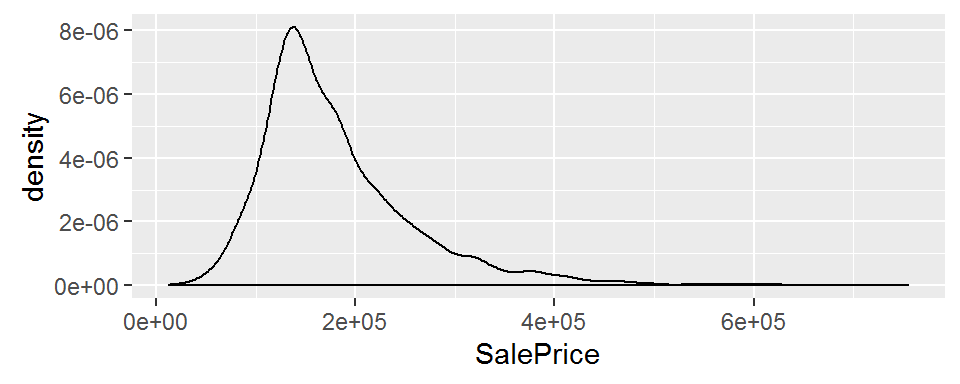In the following code, we use the dplyr package to modify the AmesHousing data; we first restrict the dataset to only houses with less than three fireplaces and then create a new variable, called Fireplace2. The as.factor command creates a factor, wich is a variable that contains a set of numeric codes with character-valued levels. Notice that the color and fill command now work properly.

# Create a new data frame with only houses with less than 3 fireplaces
AmesHousing2 <- filter(AmesHousing, Fireplaces < 3)
# Create a new variable called Fireplace2
AmesHousing2 <-mutate(AmesHousing2,Fireplace2=as.factor(Fireplaces))
#str(AmesHousing2)

ggplot(data=AmesHousing2) +
geom_density(aes(SalePrice, color = Fireplace2,  fill = Fireplace2), alpha = 0.2)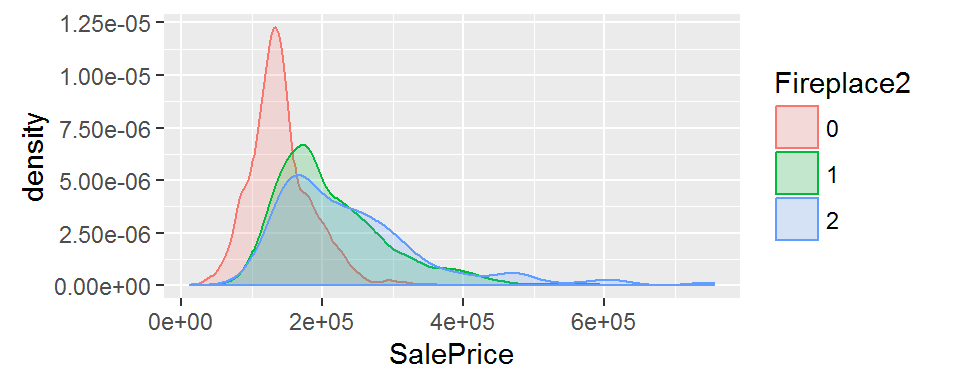Customizing graphs: In addition to using a data frame, geoms, and aes, several additional components can be added to customize each graph, such as: stats, scales, themes, positions, coordinate systems, labels, and legends. We will not discuss all of these components here, but the materials in the references section provide detailed explanations. In the code below we provide a few examples on how to customize graphs.

ggplot(AmesHousing2, aes(x = Fireplace2, y = SalePrice, color = Paved.Drive)) +
geom_boxplot(position = position_dodge(width = 1)) +
coord_flip()+
labs(title="Housing Prices in Ames, Iowa") +
theme(plot.title = element_text(family = "Trebuchet MS", color = "blue", face="bold", size=12, hjust=0))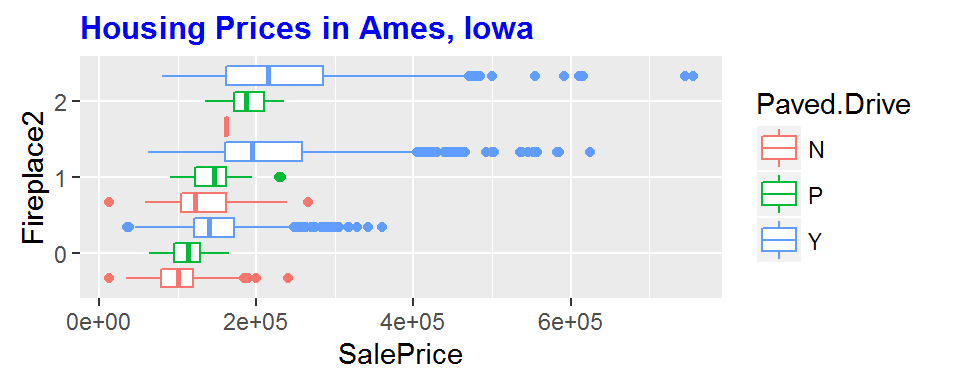Remarks:

• position is used to address geoms that would take the same space on a graph. In the above boxplot, position_dodge(width = 1) adds a space between each box. For scatterplots, position = position_jitter() puts spaces between overlapping points.
• theme is used to change the style of a graph, but does not change the data or geoms. The above code is used to modify only the title in a boxplot. A better approach for beginners is to choose among themes that were created to customize the overall graph. Common examples are theme_bw(), theme_classic(), theme_grey(), and theme_minimal(). You can also install the ggthemes package for many more options.

Questions:

1. In the density plot above, explain what the color, fill, and alpha commands are used for. Hint: try running the code with and without these commands or use the Data Visualization Cheat Sheet.

2. In the boxplot, what is done by the code coord_flip()?

3. Create a new boxplot, similar to the one above, but use theme_bw() instead of the given theme command. Explain how the graph changes.

4. Use the tab completion feature in RStudio (type theme and hit the Tab key to see various options) to determine what theme is the default for most graphs in ggplot.

7. On your own

In order to complete this activity, you will need to use the dplyr package to manipulate the dataset before making any graphics.

• Restrict the AmesHousing data to only sales under normal conditions. In other words, Condition.1 == Norm
• Create a new variable called TotalSqFt = GR.Liv.Area + Total.Bsmt.SF and remove any homes with more than 3000 total square feet.
• Create a new variable, where No indicates no fireplaces in the home and Yes indicates at least one fireplace in the home.
• With this modified data file, create a graphic involving no more than three explanatory variables that best portrays how to predict sales price. For example, Figure 12 uses a linear model of kitchen quality, above ground square footage, and number of fireplaces to predict sale price.